/

### The normal distribution

The normal distribution is a continuous probability distribution that has a bell-shaped probability density function: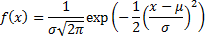It is usually considered to be the most prominent probability distribution in statistics partly because it arises in a very large number of contexts as a result of the central limit theorem and partly because it is relatively tractable analytically.

The normal distribution is also called the Gaussian distribution. The unit normal (or standard normal) distribution is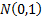.

Characteristics of the normal distribution are set out below:

 Distribution name Normal distribution Common notation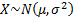Parameters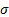= scale parameter (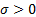)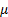= location parameter Domain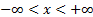Probability density function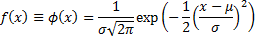Cumulative distribution function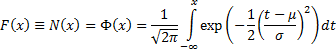MeanVariance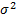Skewness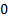(Excess) kurtosisCharacteristic function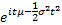Other comments The inverse unit normal distribution function (i.e. its quantile function) is commonly written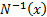(also in some texts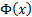and the unit normal density function is commonly written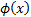.is also called the probit function.   The error function distribution is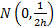, where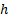is now an inverse scale parameter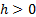.   The median and mode of a normal distribution are.   The truncated first moments of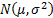are: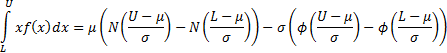whereand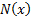are the pdf and cdf of the unit normal distribution respectively.   The mean excess function of a standard normal distribution is thus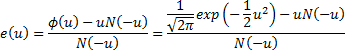The central moments of the normal distribution are: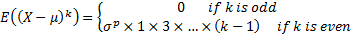Nematrian web functions

Functions relating to the above distribution may be accessed via the Nematrian web function library by using a DistributionName of “normal”. For details of other supported probability distributions see here.